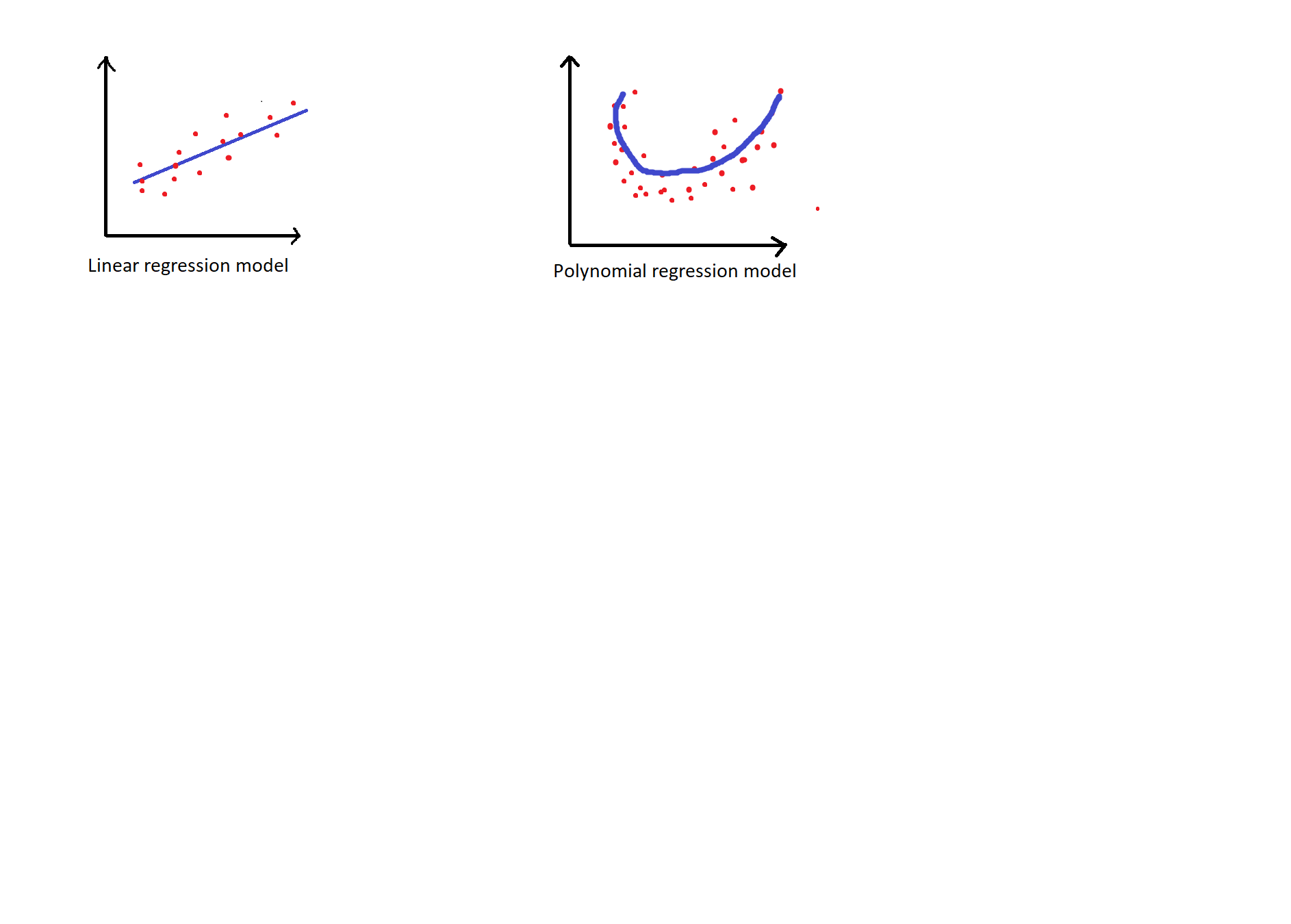# Polynomial Regression in Python

In this tutorial, we will learn Polynomial Regression in Python. We have shown the graphical representation for a better understanding.

What is polynomial regression? How is polynomial regression different from linear regression? I am now going to explain it you guys now.
Polynomial regression
It is a type of linear regression where the relationship between the independent variable and the dependent variable is modelled as an nth degree polynomial. This fits the nonlinear relationship between the independent variable and the corresponding mean of the dependent variable.

Consider the equation for linear regression:
y=a0+(Σai*xi)
here a0 is the independent variable and a1 is the dependent variable with the polynomial with degree one.

Now, this is how the polynomial regression looks like:
y=a0+(Σai*xi) +Fp

As the data that we obtain from the current world is not linear we cant use the linear model as is is not accurate. So we use the same linear model with some mapping functions like Fp to convert the model into nonlinear.
Upon adding the mapping function to the linear model it increases the accuracy of the model.

## The necessary library functions are

```import numpy as np
import matplotlib.pyplot as plt
import seaborn as sns
sns.set_style('whitegrid')

import pandas as pd
from sklearn.linear_model import LinearRegression
from sklearn.metrics import r2_score
from sklearn.preprocessing import PolynomialFeatures```

### The code to generate the plot, for the given dataset

```polynomial_regression = PolynomialFeatures(degree=2)
X_polynomial = polynomial_regression.fit_transform(X.reshape(-1, 1))
lin_reg_2 = LinearRegression()
lin_reg_2.fit(X_poly, y.reshape(-1, 1))
y_pred = lin_reg_2.predict(X_polynomial)
plt.figure(figsize=(10,8));
plt.scatter(X, y);
plt.plot(X, y_pred);
print(r2_score(y, y_pred))
```

From the above code, you can generate a fitted curve plot for the day to day dataset.

So next time if you find that your data isn’t simply linear, you can use polynomial features to get the best fit for your model to train and test the dataset.

### Here’s how the linear regression and polynomial regression model looks likeregression models

One of the advantages of the polynomial model is that it can best fit a wide range of functions in it with more accuracy.

Thanks for reading Polynomial Regression in Python, hope you are now able to solve problems on polynomial regression.